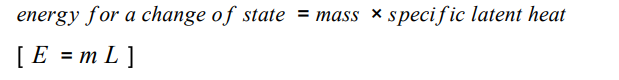# Required practical video collection for paper 2 AQA GCSE Physics Higher Tier Summer 2022 OnlyThe advanced information for AQA GCSE Physics Paper 2 Higher tier, it lists only one practical that the examiners can assess you on. Here are some videos to help – there are plenty more just search!

# AQQ GCSE Physics Changes of state and specific latent heat1. What is the energy needed for a substance to change its state called?
2. Do five star jumps.
3. When a change of state occurs, the energy supplied changes the energy stored (internal energy). What is not changed.
4. Sketch a heating curve for water. Temperature against energy supplied.
5. Define the specific latent heat of a substance.
6. Look at this equation.7. What is the unit for mass?
8. What is the unit for specific latent heat?
9. What is the unit of energy for a change of state?
10. Rearrange the equation so that m = and L =
11. What does the L refer to?
12. Define the specific latent heat of fusion.
13. Define the specific latent of vaporisation.
14. Spend twenty minutes on your Nintendo switch.
15. Sketch a heating curve and a cooling curve and label where the changes of state occur.
16. Why are the regions on the graph where there is a change of state flat?
17. What is the difference between specific heat capacity and specific latent heat?

# AQA GCSE Physics Electrical Power Focus Questions## Electrical Power Focus Questions

1. Look at these two equations to calculate electrical power.2. What is the unit of power?
3. What is the unit of potential difference?
4. What is the unit of current?
5. What is the unit of resistance?
6. In terms of energy transfer, what does a 100 W light bulb do?
7. Rearrange the first equation so that V= and I =
8. Rearrange the second equation so that R = and I =